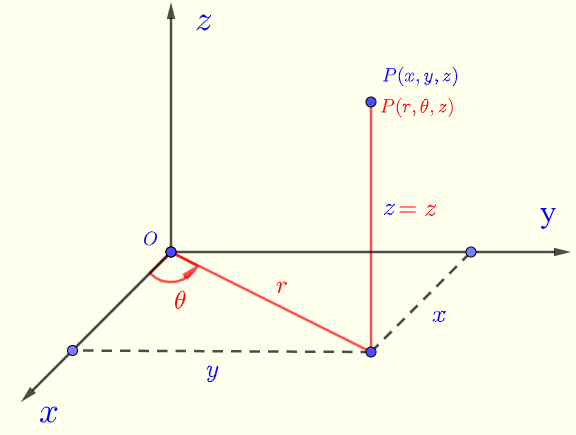# Convert Rectangular to Cylindrical Coordinates - Calculator

## Rectangular and Spherical Coordinates

Convert rectangular to spherical coordinates using a calculator.
It can be shown that the rectangular rectangular coordinates $$(x,y,z)$$ and cylindrical coordinates $$(r,\theta,z)$$ in Fig.1 are related as follows:
$$x = r \cos \theta$$ , $$y = r \sin \theta$$ , $$z = z$$       (I)
$$r = \sqrt {x^2 + y^2}$$ , $$\tan \theta = \dfrac{y}{x}$$ , $$z = z$$       (II)
with $$0 \le \theta \lt 2\pi$$Fig.1 - Rectangular and cylindrical coordinates The calculator calculates the cylindrical coordinates $$r$$ , $$\theta$$ and $$z$$ given the rectangular coordinates $$x$$ , $$y$$ and $$z$$ using the formulas in II.

## Use Calculator to Convert Rectangular to Cylindrical Coordinates

1 - Enter $$x$$, $$y$$ and $$z$$ and press the button "Convert". You may also change the number of decimal places as needed; it has to be a positive integer.
Angle $$\theta$$ is given in radians and degrees.

 $$(x , y , z )$$ = (2 , 2 , 3) Number of Decimal Places = 5 $$r = \quad$$ $$\theta = \quad$$   Radians $$\theta = \quad$$   Degrees $$z = \quad$$